Select Page

# MCQ Answers for CBSE 12 Science Maths Relations and Functions in Hindi

MCQ Answers for CBSE 12 Science Maths Relations and Functions in Hindi to enable students to get Answers in a narrative video format for the specific question.

Expert Teacher provides MCQ Answers for CBSE 12 Science Maths Relations and Functions through Video Answers in Hindi language. This video solution will be useful for students to understand how to write an answer in exam in order to score more marks. This teacher uses a narrative style for a question from Relations and Functions not only to explain the proper method of answering question, but deriving right answer too.

Please find the question below and view the Answer in a narrative video format.

Question:

## Similar Questions from CBSE, 12th Science, Maths, Relations and Functions

Question 1 : Letbe defined as f(x) = 3x. Choose the correct answer. (View Answer Video)

Question 2 : Letbe defined as. Choose the correct answer. (View Answer Video)

Question 3 : Letdefined as f(x) = x be an identity function. Then, (View Answer Video)

Question 4 :  Letdefined as f(x) = 5 be a constant function. Then its range is (View Answer Video)

Question 5 : Let A = {1, 2, 3, 4} and let R = {(2, 2), (3, 3), (4, 4), (1, 2)} be a relation on A. Then, R is, (View Answer Video)

### Application of Derivatives

Question 1 : If,then the approximate value of f(3.02) is _________. (View Answer Video)

Question 2 : Find the approximate change in volume V of a cube of side x meters caused by increasing the side by 1%. (View Answer Video)

Question 3 : Equation of normal to the curve x+y=x^y where it cuts x-axis; is (View Answer Video)

Question 4 : A balloon, which always remains spherical has a variable radius. Find the rate at which its volume is increasing with the radius when the later is 10 cm.  (View Answer Video)

Question 5 : The line y=mx+1 is a tangent to the curve ifthe value of m is ________. (View Answer Video)

### Differential Equations

Question 1 : Find the solution of the differential equation(View Answer Video)

Question 2 : Write the degree of the differential equation :(View Answer Video)

Question 3 : Write the differential equation representing the family of curves y = mx, where m is an arbitrary constant. (View Answer Video)

Question 4 : Find the general solution of differential equation(View Answer Video)

Question 5 : Write the sum of the order and degree of the differential equation(View Answer Video)

### Probability

Question 1 : Compute P(A/B) if P(B) =0.5 and P(A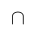B)=0.32. (View Answer Video)

Question 2 : If E and F are two events such that,, Find P(not E and not F). (View Answer Video)

Question 3 : If P(A)=0.8, P(B)=0.5 and=0.4, find. (View Answer Video)

Question 4 : If P(A)=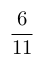, P(B)=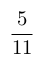and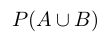=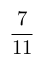, find. (View Answer Video)